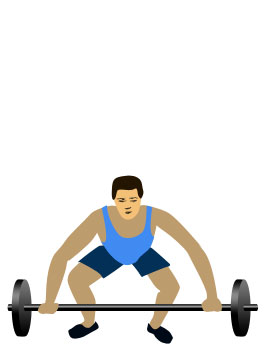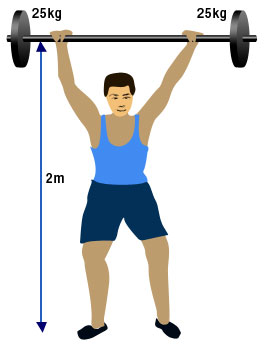## Work

When a force acts on an object and causes it to move through a distance, energy is transferred and work is done.

Work is only done when there is movement against an opposing force. For e.g. lifting a book off the floor to put on a table, here the movement is from the floor to the table and the opposing force is the weight of the book.

The amount of work done can be calculated by the equation:The unit for work is Joules (J). One joule of work is done when a force of 1 Newton moves an object through a distance of 1 metre.

 Start EndIn order to lift the barbell above his head the weight lifter need to apply a force which opposes the downward acting force of gravity on the mass of the barbell. The distance from the floor to above the lifters head is 2 metres. Mass of barbell = 25 + 25 = 50kg Weight of barbell = mass x acceleration due to gravity W = m x g W = 50 x 9.8 = 490 Newtons Work done = force x distance Work done = 490 x 2 = 980 Joules

In the above example, the work done by the weight lifter in lifting the weights was 980 joules. In order to do this work energy had to be transferred. 980 joules of chemical energy from food eaten by the weight lifter was transferred to 980 joules of gravitational potential energy to the barbell. Thus the amount of work done is equal to the energy transferred from one form to another.